﻿ Big-Bang Nucleosynthesis Reaction Rate Change Sensitivity AnalysisInternational Journal of Theoretical and Mathematical Physics

p-ISSN: 2167-6844    e-ISSN: 2167-6852

2019;  9(1): 1-8

doi:10.5923/j.ijtmp.20190901.01### Big-Bang Nucleosynthesis Reaction Rate Change Sensitivity Analysis

Niranjan Bhatia

Monta Vista High School, Cupertino, CA, USA

Correspondence to: Niranjan Bhatia, Monta Vista High School, Cupertino, CA, USA.
 Email: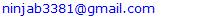Abstract

Primordial Big-Bang Nucleosynthesis (BBN) took place roughly three minutes after the big-bang and lasted for approximately 17 minutes. Light elements [D, 3He, 4He, 6Li, and 7Li] could be formed since the temperature was so high for nuclear fusion to happen. The primordial light elements abundance is related to many parameters including the thermonuclear reaction rates, temperature, baryon-to-photon ratio, and fundamental constants (e.g. gravitational constant). There are more than 88 thermonuclear reactions during the BBN out of which impact in light element abundance from rate variations is typically observed only from the 12 major BBN reactions. Variations in abundance of D/H, 3He, 7Li vary more with rate change in the reactions they are sensitive to. 4He has little sensitivity to rate variations. In this project, I am trying to analyze sensitivity of variations in primordial light element abundance to reaction rate changes using a BBN simulation software. My hypothesis is that for a particular eta value, most of variations noted in light element abundance will be due to rate scaling and slope of abundance curves over eta (due to rate changes) are expected to vary within a certain factor (10+/-2). My conclusions closely supported the hypothesis for all reactions except change in abundance of D/H in reaction d(p,γ)3He which needs further research from a nuclear physics perspective. D/H abundance change magnified with a combination of rate and eta increase. As the rate was scaled higher, steepness of D/H abundance slope increased with an increase in eta.

Keywords: Big bang nucleosynthesis, Astrophysics, Cosmology, Primordial light element abundance, Thermonuclear rates, Monte carlo simulation, D/H, 3He, 4He, 7Li, Baryon-photon ratio

Cite this paper: Niranjan Bhatia, Big-Bang Nucleosynthesis Reaction Rate Change Sensitivity Analysis, International Journal of Theoretical and Mathematical Physics, Vol. 9 No. 1, 2019, pp. 1-8. doi: 10.5923/j.ijtmp.20190901.01.

### 1. Introduction

#### 1.1. Background

Nucleosynthesis is the process which which atomic nuclei heavier than the Hydrogen atom is produced from simple nuclei by chemical reactions. A simple example is the p(n,γ)d reaction in which p and n are fused to for deuterium and emit gamma radiation: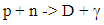(1)
The universe was born due an event called the big-bang which took place approx. 13.7 billion years ago . The big bang was an explosion which caused the expansion of the universe. At t=10-43 seconds, the temperature was 1032K and the universe was very dense. Matter and energy were inseparable. The universe started expanding and cooling. At about t=10-35 seconds, matter and energy separated in a process called baryogenesis . The expansion and cooling of the universe continued.
From t=0.01 seconds, the subatomic particles fused to form neutrons and protons. At approx. t=1 sec, the particles could fused to form the heavier nuclei of D, He, and Li but the temperature was so high that the heavier nuclei could not survive due to collisions with high-energy photons created as a result of collisions between the subatomic particles .
As the temperature further cooled, the atomic nuclei started forming and surviving. Thus, the light elements (D, 3He, 4He, 6Li, 7Li) were formed in an event known as the Primordial BBN which took place approx. three minutes after the big-bang [1, 9, 10]. These elements could be formed since the temperature was high enough (109K) for nuclear fusion to take place [1, 3].
There are more than 88 thermonuclear reactions which took place during BBN. Out of them, 12 are the most important as they impact the light element abundance for 4He, D, 3He, and 7Li . The 12 reactions are n−decay, p(n,γ)d, d(p,γ)3He, d(d,n)3He, d(d,p)t, 3He(n,p)t, t(d,n)4He, 3He(d,p)4He, 3He(α,γ)7Be, t(α,γ)7Li, 7Be(n,p)7Li, and 7Li(p,α)4He . The BBN light element abundances BBN are impacted by reaction rates uncertainties as well as the baryon-photon ratio (eta) .
BBN lasted approx. 17 minutes . After that, the temperature cooled and dropped below the requirement for nuclear fusion preventing the formation of the heavier elements (including metals). They were later formed due to the stellar nucleosynthesis [1, 3, 11]. Stellar nucleosynthesis is the process by which light nuclei fuse to form heavier ones as a result of nuclear reactions occurring in the stars . The first stars and galaxies were formed approx. 300 - 500 million years after the big bang .
The conditions during the BBN are believed to be well described Standard BBN model for determining values including temperature, expansion rate, nucleon density, and neutrino content . The primordial abundances depend on the thermonuclear reaction rates as well as on other parameters including temperature, baryon-to-photon ratio, and fundamental constants . The thermonuclear reaction rates are important inputs for modeling BBN . The baryon-photon ratio (η = 2.74 * 10-8 Ωbh2 = 6.11±0.04 * 10-10 where h is the Hubble constant and Ωb is the baryonic density) is an important parameter determining the primordial light element abundances and is the ratio of the number density of baryons to the number density of photons .
3He abundance has changed little since the evolution of the universe. Variations in thermonuclear reaction rates are important in determining the D/H abundance. The abundance of D/H is sensitive to the rates of the following five reactions: n<->p, p(n,γ)d, d(d,n)3He, 3He(d,p)4He, d(d,p)t . Overall, 3He is fairly sensitive to rates of six major reactions, has low sensitivity to two reactions, and no sensitivity to four reactions .
The abundance of 7Li is higher by a factor of ∼3 when calculated theoretically via the BBN vs the abundance found in the universe [15, 16, 17, 18, 7, 11]. 7Li is fairly sensitive to rates of eight major reactions and has low sensitivity to four reactions . Estimations on 4He abundance will have no impact in any "strong" BBN nuclear reaction since it depends on the neutron lifetime which equivalently is the strength of a weak interaction [7, 19].
The nuclear reaction rates are important inputs for modeling BBN and stellar evolution . Compared to BBN, totally different codes are involved in studying stellar production of elements. The values of BBN thermonuclear reaction rate are entered as thermal rates [14, 6]. My research builds on top of the existing work by further researching how the primordial light element abundance changes with the increase in reaction rates or whether there are other factors impacting it?
Based on existing published research, the impact in light element abundance will be observed only from rate variations of the 12 major BBN reactions . Variation of final abundance with baryon-photon ratio (eta) has no analytical solution and is very complex.
Also, for an eta value, variation of final abundance with input reaction rate will not be linear due to the coupled nature of system. That is, with value of abundance at a given time step t(n) in simulation, set of reaction rates that interconnect these abundances, temperature, and density of system as inputs, the solution for abundances at next time step t(n)+dt is found by solving a system of first-order coupled differential equations.
Reactions have different sensitivities to the reaction rates. Variations in abundance of D/H, 3He, 7Li will vary more with rate change in the reactions they are sensitive to . There should be little sensitivity of 4He to rate .

#### 1.2. Model Parameters

Eta is a free parameter in the standard BBN model, that is, it’s value is not predefined but is chosen or adjusted. All the abundances change with eta. Hence, it is an independent variable in our simulations because its value is changed by the system while running the different iterations of the simulations. The reaction rates are input variables into the model. Once the reaction rates are input for certain reactions, they cannot be modified by the system between the different iterations. To modify the rates, the rate would need to be re-entered and the simulations would need to be re-executed.
Since eta is independent variable and reaction rate the input, variations will be noted from both . Hence, in this project we modify the reaction rates which is the model input parameter with eta being the independent variable and observe changes in the abundance which is the dependent variable or output.

#### 1.3. Hypothesis

In this project, I am trying to analyze sensitivity of variations in primordial light element abundance to reaction rate changes using a BBN simulation software. My hypothesis is that for a particular eta value, most of variations noted in light element abundance will be due to rate scaling and slope of abundance curves over eta (due to rate changes) are expected to vary within a certain factor (10+/-2) .

### 2. Materials & Methods

#### 2.1. Project Methods

My general project methods are computational simulation, sensitivity study, and what-if scenario analysis.

#### 2.2. Software

Bigbangonline.org (Kawano code engine) BBN simulation software was used for the project since its features are perfectly compatible with the project goals. It takes thermonuclear reaction rates as inputs, runs simulations, and produces the predictions of light element abundances. Its engine runs in the cloud. The only software installation needed to run it is a light-weight interface.

#### 2.3. Model Variables

The model input variable was the thermonuclear reaction rate which was varied for 11 of the 12 major BBN thermonuclear reactions. Sample of other 66 non-major reactions are taken. The independent variable was the baryon-photon ratio. The baryon–photon ratio is a key parameter determining the primordial light element abundance. The control variables were reactions, nuclides, fundamental constants (including the gravitational constant and cosmological constant), the neutron lifetime, number of neutrino species, and the temperature. The dependent variable was the final predicted primordial light element abundances. The primordial light element abundance is the measure of the present quantity of the light elements D, 3He, 4He, and 7Li from the Big Bang.
The standard BBN model makes predictions of light element abundances formed very early in the Universe, with one free parameter (baryon-photon ratio or eta). These are compared to observations made today, and the values of eta are found that give consistency between predictions and observations. Light elements were produced during the BBN, while elements heavier than helium were formed due to stellar nucleosynthesis which occurred much later.

#### 2.4. Process

The BBN simulation software used can be downloaded and launched from http://www.bigbangonline.org/. The reaction rates can be modified in the menu option “Nuclear Reaction Rates and Rate Libraries” -> “Rate Manager”. The BBN simulations can be performed by selecting the appropriate thermonuclear reaction rate for the reaction and setting the values of the parameters including “Computational Parameters”, “Early Universe Parameters”, “Looping Parameters”, and number of trials in the Monte Carlo Simulation.
Once the simulations are complete, the simulation results can be visualized by clicking on the option “Final Abundance Plotting Interface” and seeing the details on the primordial abundances of the light elements. The results can be reviewed and compared to the results with default parameter values to determine variations in the abundance of the light elements. The whole process can be repeated by modifying different reaction rates as well as creating a new rate.

### 3. Results

The simulation variable values were as follows:
 Table 1. Simulation variable values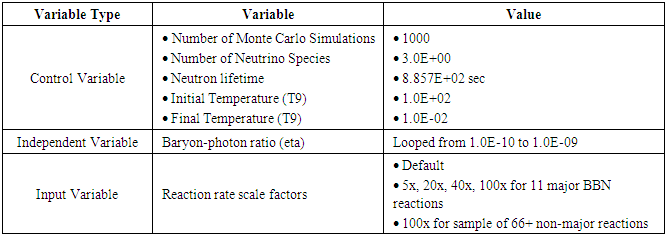The values of the dependent variable, viz., light element abundance, were observed. Initially, simulations were executed with default parameter values and the plot of abundance values was noted (Figure 1).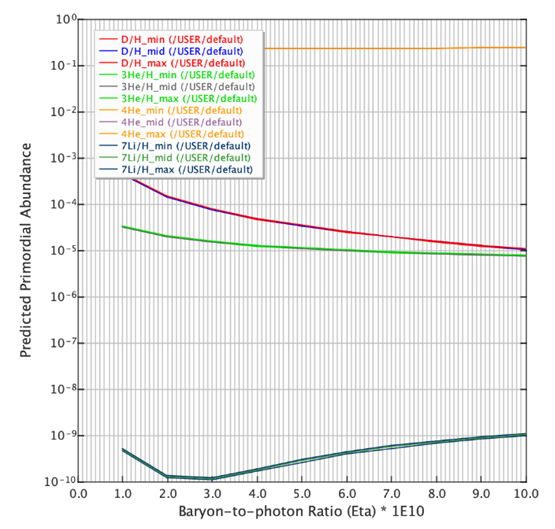Figure 1. Abundance plot with default parameter values
Then the simulations were run with run with rate changes by varying the rates of the BBN reactions 5x, 20x, 40x, and 100x. As expected, variations in light element abundance with rate changes were noted for all 11 major reactions (Figure 2, 3, 4, 5). Sample of nine non-major reactions had insignificant impact on light element abundance with maximum rate change scaling factor 100x. Hence, other scaling factors were not considered.
For D/H abundance, rate sensitivity was noted in four reactions: d(p,γ)3He (Figure 2), d(d,n)3He (Figure 3a), d(d,p)t (Figure 3c), p(n,γ)d (Figure 3d). The D/H abundance in the three reactions d(d,n)3He, d(d,p)t, p(n,γ)d changed within a factor of 10+/-2. For the fourth reaction d(p,γ)3He, D/H abundance change had a much higher decrease of slope with eta. We can also note that the rate variations increased as eta increased.
Figure 2 shows the plot of rate variations for the reaction d(p,γ)3He. From the plot, we can note that for eta 2.0, the abundance varied by a factor 10-4. But for eta 10.0, it varied by a factor 10-10. As rate was scaled higher, steepness of slope of D/H abundance increased with eta until a certain value, and then decreased. With rate increase, the value of eta at which steepness of D/H decreased also decreased.
From figure 2a, we can also note that for the reaction d(p,γ)3He with rate change scaling factor 5x, the D/H abundance ranged from 10-4 to 10-7. Compared to the default values, D/H slope decreased from eta 6.0. Figure 2b shows that for rate change scaling factor 20x, the D/H abundance ranged from 10-4 to 10-11. The D/H abundance slope increased (compared to rate 5x) from eta 2.0, then decreased from eta 8.5. Figure 2c shows that for rate change scaling factor 40x, the D/H abundance ranged 10-4 to 10-13. The D/H abundance slope increased (compared to rate 20x) from eta 2.0 but then decreased from eta 5.0. Figure 2d shows that for rate change scaling factor 100x, the D/H abundance ranged 10-4 to 10-16. Hence, we can note that the D/H abundance slope increased (compared to rate 40x) from eta 1.0 but then decreased from eta 3.0.
For 3He, the abundance increased/decreased by a factor less than 100 with reaction rate change for the following reactions: 3He(d,p)4He (Figure 3b), d(d,p)t (Figure 3c), 3He(n,p)t (Figure 4a), d(p,γ)3He (Figure 2), and d(d,n)3He (Figure 3a). There was no observable impact for 4He abundance (Figure 2, 3, 4, 5). For 7Li abundance, fractional change was observed in two of the 11 major reactions (Figure 3c, 4a). Greater impact (less than a factor of 100) was observed in nine of the 11 major reactions (Figure 2, 3a-b, 3d, 4b-d, 5a-b).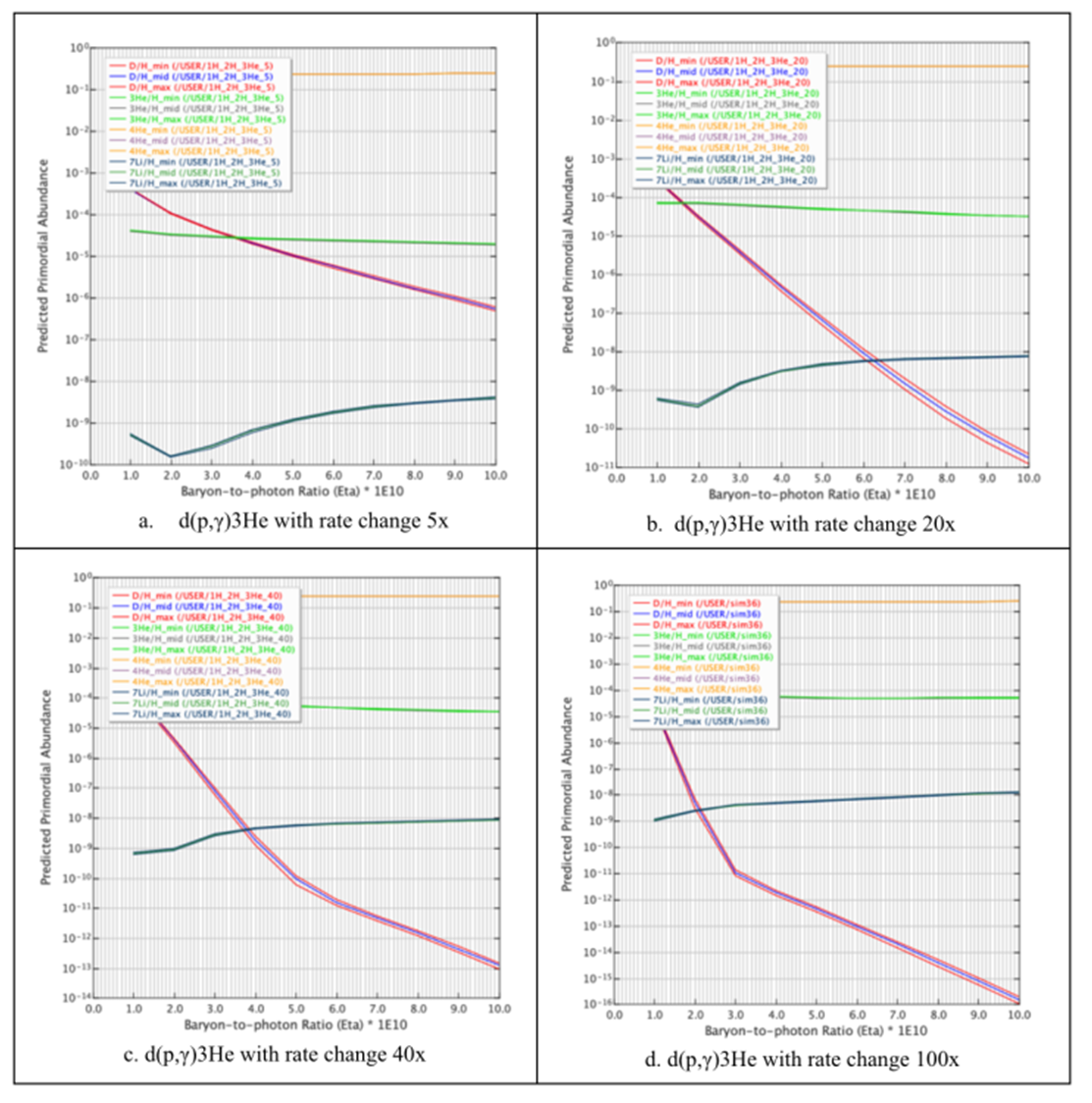Figure 2. Abundance plot with rates changes 5x, 20x, 40x, 100x for d(p,γ)3He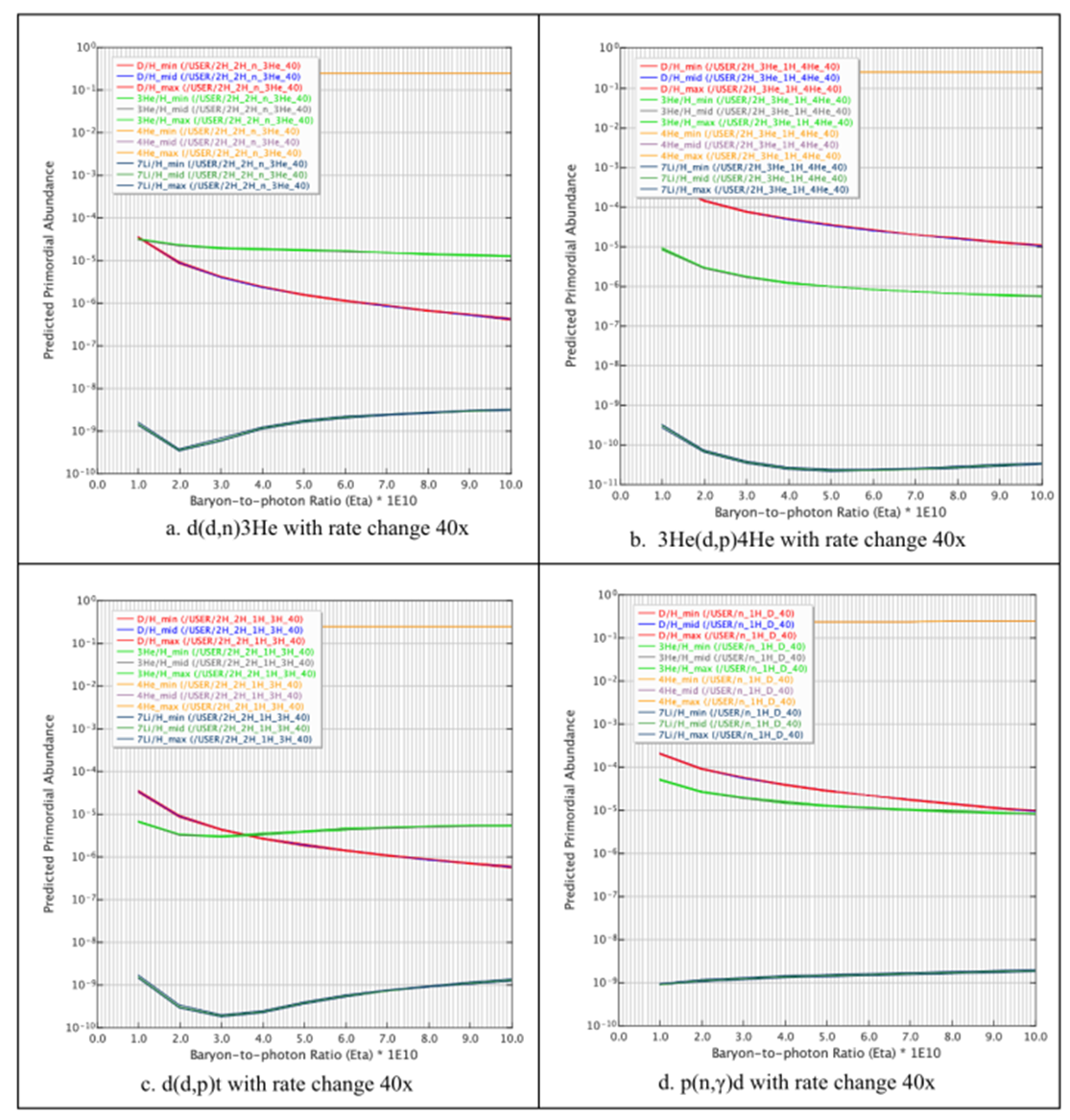Figure 3. Abundance plots with rate change 40x for d(d,n)3He, 3He(d,p)4He, d(d,p)t, & p(n,γ)d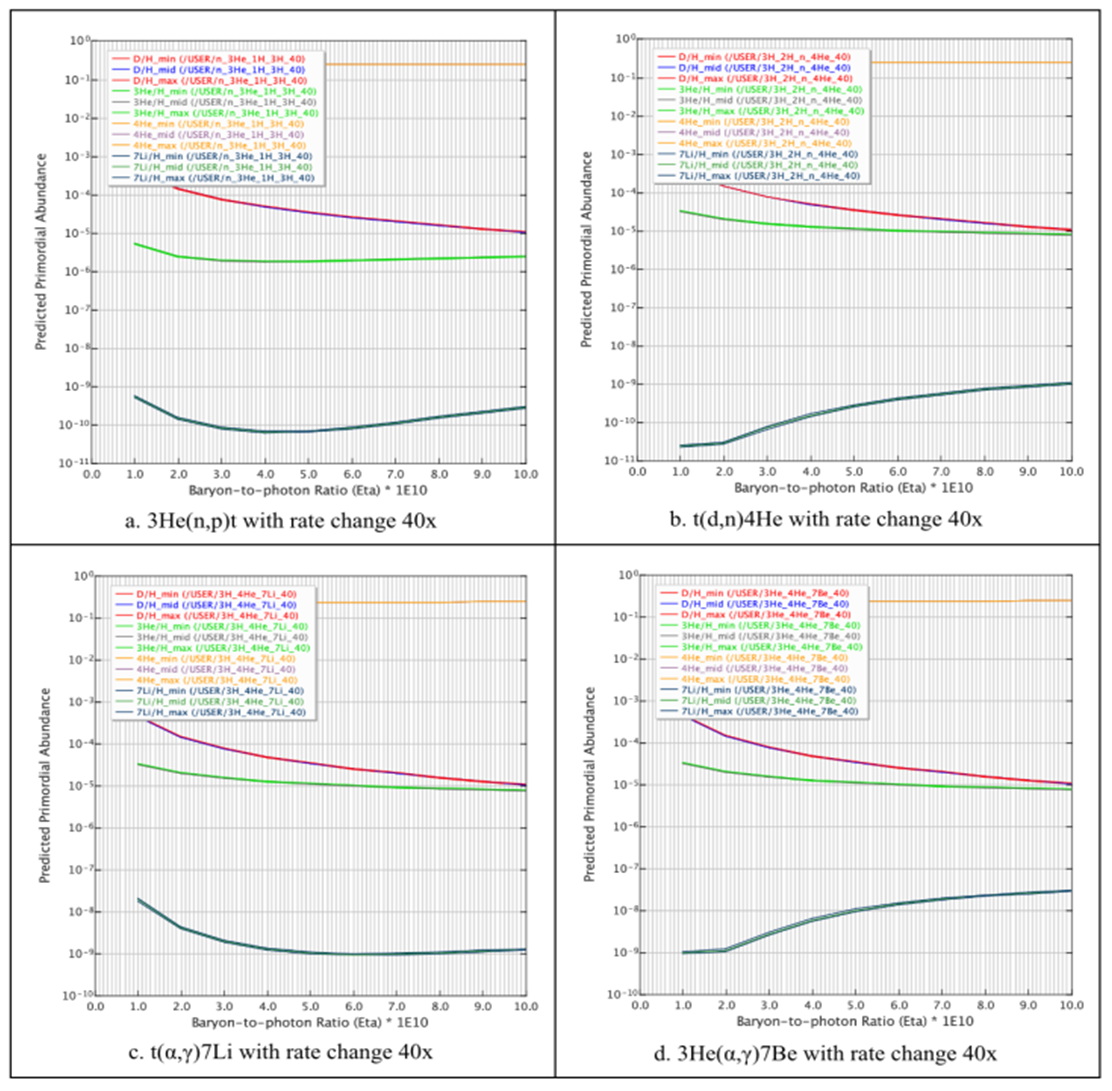Figure 4. Abundance plot with rate change 40x for 3He(n,p)t, t(d,n)4He, t(α,γ)7Li, & 3He(α,γ)7Be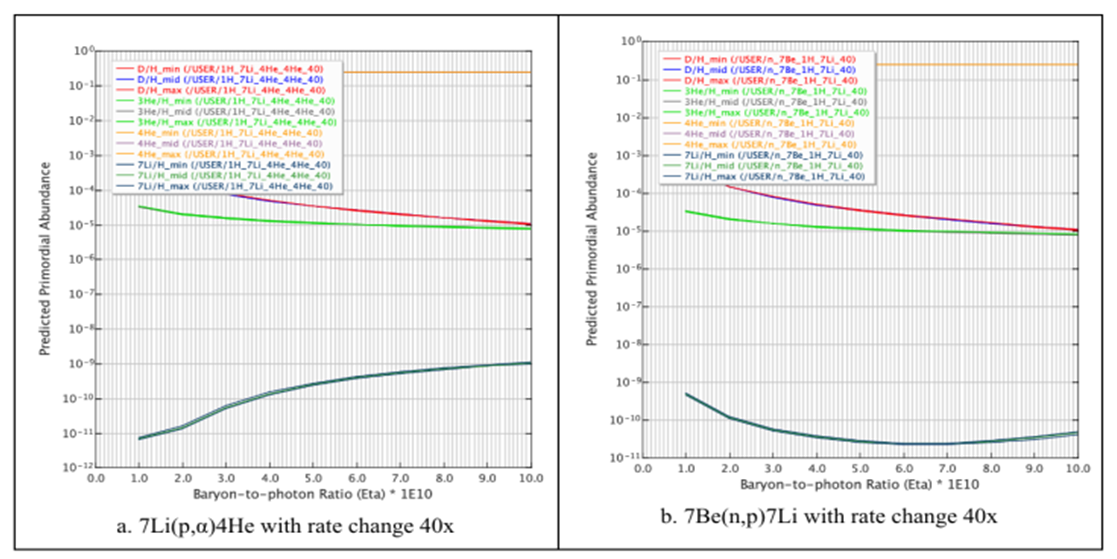Figure 5. Abundance plot with rate change 40x for 7Li(p,α)4He & 7Be(n,p)7Li
Table 2 below summarizes the results of the major BBN reaction simulations. Please note that the plots of the various reactions need to be compared to the plot for the simulation with default parameter values in Figure 1.
 Table 2. Summary results of major BBN reaction simulations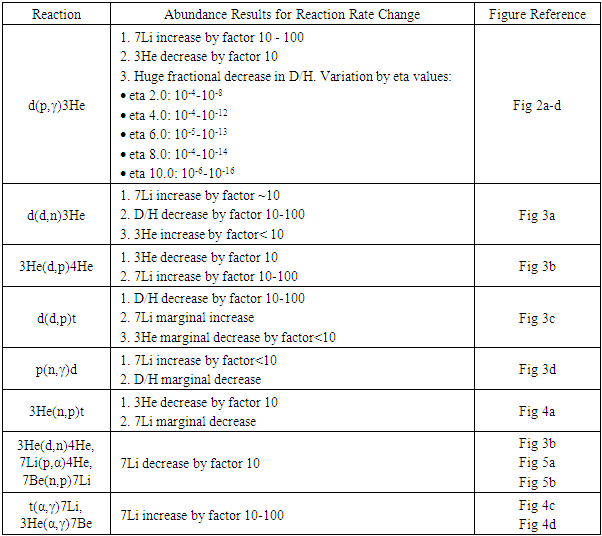### 4. Discussion

As expected, variations in light element abundance were noted when rates of all 11 major reactions were modified.
For D/H abundance, changes were observed in 4 reactions: d(p,γ)3He, d(d,n)3He, d(d,p)t, p(n,γ)d. Abundance results for 3 reactions d(d,n)3He, d(d,p)t, and p(n,γ)d changed by factor 10+/-2 with rate increase. The fourth reaction, d(p,γ)3He, showed a steep decline in D/H slope with eta with rate change increase. The change in abundance magnified with a combination of rate and eta increase. For eta 2.0, the abundance varied by a factor of 10-4 with rate. But for eta 10.0, it varied by a factor of 10-10 with rate. As rate was scaled higher, the steepness of slope of D/H abundance increased with eta until a certain value, and then decreased. With increase in rate, the value of eta at which steepness decreased also decreased.
For 3He abundance, as expected, fractional change with rate variations was noted in the five reactions: 3He(d,p)4He, d(d,p)t, 3He(n,p)t, d(p,γ)3He, and d(d,n)3He. For 7Li abundance, marginal change was observed with rate variations in 7Li abundance in two major reactions. Greater fractional impact (within a factor of 10+-2) was observed in nine of 11 reactions. For 4He abundance, as expected, there was no impact to 4He abundance (see research section for details).
Overall, the results of all reactions except d(p,γ)3He supported the hypothesis, viz., the slope of abundance curves over eta changed by factor within 10+/-2 and variations noted for a particular eta value were due to rate change. The exception noted was D/H abundance in reaction d(p,γ)3He. For this reaction, the rate variations increased with an increase in eta and the magnitude of change was much greater than the factor of 10+/-2 (from 10-4 with eta 2.0 to 10-10 for eta 10.0). As rate was scaled higher, D/H abundance sharply declined with increase in eta. Hence, the D/H abundance shows sensitivity to a combination of rate change as well as eta. Hence, the results support the hypothesis for all reactions except D/H abundance for reaction d(p,γ)3He which needs further investigation from nuclear physics perspective.
However, there are limitations. The simulation is only a tool which codifies our knowledge of the process of formation of light elements, takes input variable values, and produces output. BBN abundance depends on other factors including fundamental constants and expansion rate. To get a holistic picture, other factors also need to be explored. In this simulation, one independent variable, baryon-photon ratio (eta), was modified. Since reactions are dependent on multiple variables, impact of modifying other variables like temperature and fundamental constants also needs to be accounted for.
Accurate knowledge of reaction rates is important in nuclear astrophysics for understanding energy generation. My work will help to understanding rate change impacts to guide future nuclear physics measurements. My work has certainly inspired me to ask more questions about impact of other factors on BBN including fundamental constants, magnetic fields, and dark matter.

### ACKNOWLEDGEMENTS

I wish to thank my physics teacher at Monta Vista High School, Mr. Jim Birdsong, for helping me and keeping me motivated to complete this research work which was submitted to the Google Science Fair in 2018.

### References

  White, Martin, Professor of Physics & Astronomy, UC Berkeley. “Big Bang Nucleosynthesis.” http://w.astro.berkeley.edu/~mwhite/darkmatter/bbn.html. Accessed Jan 05, 2019.  “Timeline of the Big Bang.” The Physics of the Universe. www.physicsoftheuniverse.com/topics_bigbang_timeline.html. Accessed Jan 5, 2019.  Pagel, B.E.J., “Nucleosynthesis and Chemical Evolution of Galaxies.”, Cambridge University Press., ISBN 0 521 55958 8, 1997.  Mathews, G., et. al. “Introduction to Big Bang Nucleosynthesis and Modern Cosmology.” International Journal of Modern Physics E. 26. 10.1142/S0218301317410014, 2017.  Smith, Michael, et. al., “Experimental, computational, and observational analysis of primordial nucleosynthesis”, The Astrophysical Journal Supplement Series, 85. 219-247. 10.1086/191763, 1993.  Cyburt, Richard H., et. al., “The NACRE Thermonuclear Reaction Compilation and Big Bang Nucleosynthesis”, New Astron., 6:215-238, 2001.  Coc, Alain, et. al., “Recent results in nuclear astrophysics”, Eur. Phys. J. A, 51: 34, 2015.  Fields, B.D., et. al. “Big-Bang Nucleosynthesis.” Chin. Phys. C38, 339-344, 2014.  “Big Bang Nucleosynthesis: Cooking up the first light elements”, Einstein online. http://www.einstein-online.info/spotlights/BBN.html. Accessed Jan 05, 2019.  Sarkar, S., “Big Bang nucleosynthesis and physics beyond the Standard Model.” Rept.Prog.Phys.59, 1996, 1493-1610.  Henry, J., “The elements of the universe point to creation”, Journal of Creation, 20(2), 2006, 53–60.  Clayton, Donald G., “Principles of Stellar Evolution and Nucleosynthesis”, New York: McGraw-Hill, 1986.  Mathews, G., et. al. “Introduction to Big Bang Nucleosynthesis and Modern Cosmology.” International Journal of Modern Physics E. 26. 10.1142/S0218301317410014, 2017.  Mishra, Abhishek, Basu, D. N. “Nuclear reaction rates and the primordial nucleosynthesis.” Rom.J.Phys.57, 2012, 1317-1327.  Singh, V., et. al.,. “Primordial lithium abundance problem of BBN and baryonic density in the universe.” arXiv: 1708.05567v2 [nucl-th], 2007.  Broggini, C., et. al., “The cosmological 7Li problem from a nuclear physics perspective”, Journal of Cosmology and Astroparticle Physics - JCAP, 2012, 10.1088/1475-7516/2012/06/030.  Scherrer, R. T., et. al., “Big bang nucleosynthesis with stable 8Be and the primordial lithium problem”, Phys. Rev. D 96, 083507, 10/2017.  Bertulani, C.A., Shubhchintak, Mukhamedzhanov, A.M. “Cosmological Lithium Problems.” IX European Summer School on Experimental Nuclear Astrophysics, St. Tecla (Catania), Italy, 17-24/Sept/2017.  Kurki-Suonio, H., “Big Bang Nucleosynthesis”, 53755 Special course in cosmology lecture notes, http://www.helsinki.fi/~hkurkisu/cpt/Cosmo7.pdf. Accessed Jan 05, 2019.